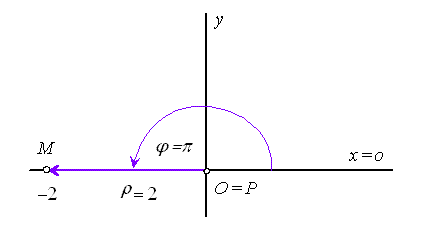Example 2. Find Cartesian coordinates of point determined by polar coordinates $M=\left(2,\pi \right)$.

Solution. Cartesian coordinate system is determined by $\left(O=P,\phantom{\rule{2mm}{2mm}}x=\stackrel{\to }{o},\phantom{\rule{2mm}{2mm}}S\in y,\phantom{\rule{2mm}{2mm}}y\perp x\right)$, Cartesian coordinates of point M are in the form M= $\left(x,y\right)$, where

 $x=\rho \mathrm{cos}\phantom{\rule{mediummathspace}{0.2em}}\varphi =2\mathrm{cos}\phantom{\rule{mediummathspace}{0.2em}}\pi =-2$
 $y=\rho \mathrm{sin}\phantom{\rule{mediummathspace}{0.2em}}\varphi =2\mathrm{sin}\phantom{\rule{mediummathspace}{0.2em}}\pi =0$

and

 $M=\left(-2,0\right)$Figure 1: Cartesian coordinates of point M